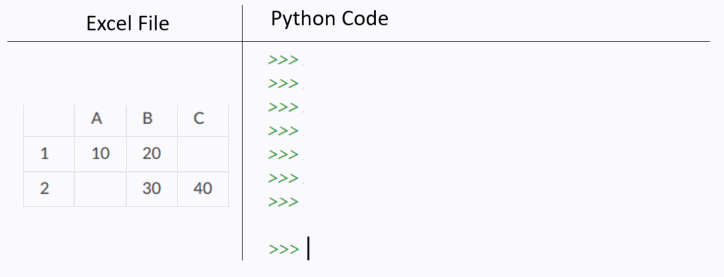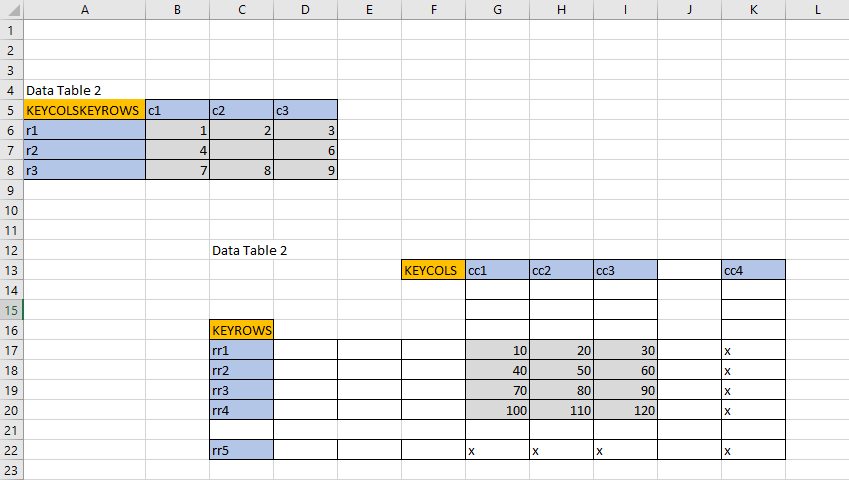# 2. Quick Start Guide¶

Get up and running in less than 5 minutes with pylightxl!Read a csv file with contents:

```import pylightxl as xl

# set the delimiter of the CSV to be the value of your choosing
# set the default worksheet to write the read in CSV data to

# make modifications to it then,
# now write it back out as a csv; or as an excel file, see xl.writexl()
xl.writecsv(db=db, fn='new.csv', ws=('sh2'), delimiter=',')
```

```import pylightxl as xl

# readxl returns a pylightxl database that holds all worksheets and its data

# pylightxl also supports pathlib as well
my_pathlib = pathlib.Path('folder1/folder2/excelfile.xlsx')

# pylightxl also supports file-like objects for django users
with open('excelfile.xlsx', 'rb') as f:

# return all sheetnames
db.ws_names
>>> ['Sheet1', 'Sheet3']
```

## 2.3. Access Worksheet and Cell Data¶

The following example assumes `excelfile.xlsx` contains a worksheet named `Sheet1` and it has the following cell content:

 A B C 1 10 20 2 30 40

```db.ws(ws='Sheet1').address(address='A1')
>>> 10
# access the cell's formula (if there is one)
>>> ''
# access the cell's comment (if there is one)
>>> 'this is a comment on cell A1!'
# note index a empty cell will return an empty string
>>> ''
# however default empty value can be overwritten for each worksheet
db.ws(ws='Sheet1').set_emptycell(val=0)
>>> 0
```

### 2.3.2. Via Cell Index¶

```db.ws(ws='Sheet1').index(row=1, col=2)
>>> 20
# access the cell's formula (if there is one)
db.ws(ws='Sheet1').index(row=1, col=2, output='f')
>>> '=A1+10'
# note index a empty cell will return an empty string
db.ws(ws='Sheet1').index(row=100, col=1)
>>> ''
# however default empty value can be overwritten for each worksheet
db.ws(ws='Sheet1').set_emptycell(val=0)
db.ws(ws='Sheet1').index(row=100, col=1)
>>> 0
```

### 2.3.3. Via Cell Range¶

```db.ws(ws='Sheet1').range(address='A1')
>>> 10
>>> [[10, 20, ''], ['', 30, 40]]
# get the range's formulas
>>> [['=10', '=A1+10']]
# update a range with a single value
```

### 2.3.4. Get entire row or column¶

```db.ws(ws='Sheet1').row(row=1)
>>> [10,20,'']

db.ws(ws='Sheet1').col(col=1)
>>> [10,'']
```

### 2.3.5. Iterate through rows/cols¶

```for row in db.ws(ws='Sheet1').rows:
print(row)

>>> [10,20,'']
>>> ['',30,40]

for col in db.ws(ws='Sheet1').cols:
print(col)

>>> [10,'']
>>> [20,30]
>>> ['',40]
```

### 2.3.6. Update Cell Value¶

```db.ws(ws='Sheet1').address(address='A1')
>>> 10
>>> 100

db.ws(ws='Sheet1').update_index(row=1, col=1, val=10)
db.ws(ws='Sheet1').index(row=1, col=1)
>>> 10
```

### 2.3.7. Update Cell Formula¶

Same as update cell value except the entry must begin with a equal sign “=”

Note

updating a cell formula will clear the previously read in cell value. Formulas will not calculate their cell value until the excel file is opened.

```db.ws(ws='Sheet1').update_address(address='A1', val='=B1+100')
db.ws(ws='Sheet1').update_index(row=1, col=1, val='=B1+100')
```

### 2.3.8. Get Named Ranges¶

```# define a named range
# get the contents of a named ranges
db.nr(name='Table1')
>>> [[10, 20], ['', 30]]
# find the location of a named range
db.nr_loc(name='Table1')
>>> ['Sheet1','A1:B2']
# update the value of a named range
db.update_nr(name='Table1', val=10)
# see all existing named ranges
db.nr_names
>>> {'Table1': 'Sheet1!A1:B2'}
# remove a named range
db.remove_nr(name='Table1')
```

### 2.3.9. Get row/col based on key-value¶

Note: key is type sensitive

```# lets say we would like to return the column that has a cell value = 20 in row=1
db.ws(ws='Sheet1').keycol(key=20, keyindex=1)
>>> [20,30]

# we can also specify a custom keyindex (not just row=1), note that we now are matched based on row=2
db.ws(ws='Sheet1').keycol(key=30, keyindex=2)
>>> [20,30]

# similarly done for keyrow with keyindex=1 (look fora match in col=1)
db.ws(ws='Sheet1').keyrow(key='', keyindex=1)
>>> ['',30,40]
```• note that `ssd` function takes any key-word argument as your KEYROWS/KEYCOLS flag
• multiple tables are read the same way as you would read a book. Top left-to-right, then down
```import pylightxl

# request a semi-structured data (ssd) output
ssd = db.ws(ws='Sheet1').ssd(keycols="KEYCOLS", keyrows="KEYROWS")

ssd
>>> {'keyrows': ['r1', 'r2', 'r3'], 'keycols': ['c1', 'c2', 'c3'], 'data': [[1, 2, 3], [4, '', 6], [7, 8, 9]]}
ssd
>>> {'keyrows': ['rr1', 'rr2', 'rr3', 'rr4'], 'keycols': ['cc1', 'cc2', 'cc3'], 'data': [[10, 20, 30], [40, 50, 60], [70, 80, 90], [100, 110, 120]]}
```

## 2.5. Write out a pylightxl.Database as an excel file¶

Pylightxl support excel writing without having excel installed on the machine. However it is not without its limitations. The writer only supports cell data writing (ie.: does not support graphs, formatting, images, macros, etc) simply just strings/numbers/equations in cells.

Note that equations typed by the user will not calculate for its value until the excel sheet is opened in excel.

```import pylightxl as xl

# read in an existing worksheet and change values of its cells (same worksheet as above)
# overwrite existing number value
db.ws(ws='Sheet1').index(row=1, col=1)
>>> 10
db.ws(ws='Sheet1').update_index(row=1, col=1, val=100)
db.ws(ws='Sheet1').index(row=1, col=1)
>>> 100
# write text
db.ws(ws='Sheet1').update_index(row=1, col=2, val='twenty')
# write equations

xl.writexl(db=db, fn='updated.xlsx')
```

## 2.6. Write a new excel file from python data¶

For new python data that did not come from an existing excel speadsheet.

```import pylightxl as xl

# take this list for example as our input data that we want to put in column A
mydata = [10,20,30,40]

# create a blank db
db = xl.Database()

# add a blank worksheet to the db